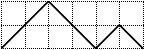Queries for Dyck paths: search statistic / browse statistics / browse maps from / browse maps to

# Definition & Example

• A Dyck path of semilength $n$ is a lattice path from $(0,0)$ to $(2n,0)$ consisting of $n$ up steps of the form $(1,1)$ and $n$ down steps of the form $(-1,1)$ which never goes below the x-axis $y=0$. Denote all Dyck paths of size $n$ by $\mathfrak{D}_n$.

Equivalently, a Dyck path of semilength $n$ can be seen as

• a lattice path from $(0,0)$ to $(n,n)$ consisting of $n$ up steps of the form $(1,0)$ and $n$ down steps of the form $(0,1)$ which never goes below the diagonal $y=x$.

• a Dyck word, this is a word in $\{0,1\}$ such that any prefix contains at least as many $1$'s as it contains $0$'s. Seeing a $1$ as an opening bracket and a $0$ as a closing bracket, Dyck words can be seen as well-formed bracketing systems.

 the 5 Dyck paths of size 3[1,0,1,0,1,0] [1,0,1,1,0,0] [1,1,0,0,1,0] [1,1,0,1,0,0] [1,1,1,0,0,0]

• There are $\operatorname{Cat}(n) = \frac{1}{n+1}\binom{2n}{n}$ Dyck paths of semilength $n$, see OEIS:A000108. This can for example be seen using the reflection principle.

# Properties

• A Dyck path $D$ of size $n+1$ can be decomposed into a Dyck path $D_1$ of size $k$ and a Dyck path $D_2$ of size $n-k$, where

• $(2k+2,0)$ is the first touch point of $D$ at the x-axis,

• $D_1$ is the prefix of $D$ from $(1,1)$ to $(2k-1,1)$ never going below the line $y=1$, and where

• $D_2$ is the suffix of $D$ from $(2k,0)$ to $(2n,0)$.

This yields the recurrence

$$\operatorname{Cat}(n+1) = \sum_{k=1}^n \operatorname{Cat}(k) \cdot \operatorname{Cat}(n-k).$$

# Remarks

• There is a natural extension of Dyck paths to $m$-Dyck paths which can be seen as lattice paths from $(0,0)$ to $(mn,n)$ that never go below the diagonal $y = \frac{1}{m} x$. The number of $m$-Dyck paths is given by the Fuss-Catalan number $C^{(m)}(n) = \frac{1}{mn+1} \binom{(m+1)n}{n}$ [Kra89].

• Dyck paths and $m$-Dyck paths can be seen as type A instances of a more general combinatorial object for root systems, namely positive chambers in the (generalized) Shi arrangement [Ath04].

• Dyck paths of semilength $n$ are in canonical bijection with Nakayama algebras with linear quiver and $n+1$ simple modules.

• [Ath04] C.A. Athanasiadis, Generalized Catalan numbers, Weyl groups and arrangements of hyperplanes, Bull. London Math. Soc., 36 (2004), pp. 294-392
• [Kra89] C. Krattenthaler, Counting lattice paths with a linear boundary II, Sitz.ber. d. ÖAW Math.-naturwiss. Klasse 198 (1989), 171-199

# Technical information for database usage

• A Dyck path is uniquely represented as a Dyck word.
• Dyck paths are graded by the semilength.
• The database contains all Dyck paths of semilength at most 8.

If you want to edit this wiki page, you can download the raw markdown and send your new version to info@findstat.org